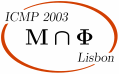ICMP 2003 > Sessions > General relativity

# General relativity

Session organized by A. Rendall (Potsdam), R. Bartnik (Canberra).

## Talks

 P. Bizon Cracow H. Bray MIT P. Chrusciel Tours H. Ringstrom Max Planck I. Rodnianski Princeton E. Seidel Max Planck

P. Bizon
Institute of Physics
Jagellonian University
Reymonta 4
30-059 Krakow
Poland

Formation of singularities for Yang-Mills equations

We report on recent studies of singularity formation in solutions of Yang-Mills equations in higher dimensions with emphasis on the five dimensional case which exhibits interesting similarities with the Einstein equations in the physical dimension.

Hubert Bray
MIT

Estimates on the ADM mass using inverse mean curvature-like flows

In 1997, Huisken and Ilmanen showed that the ADM mass of a totally geodesic, asymptotically flat, space-like slice M of a spacetime is bounded below by the Hawking mass of any surface S in M which is not enclosed by a surface with less area in M. Following the work of Geroch, Jang, and Wald, they showed that it was always possible to flow such a surface in M by a "generalized" inverse mean curvature flow in such a way that the Hawking mass is nondecreasing and converges to the ADM mass for a large round sphere at infinity. In the current talk we consider the question of finding other flows under which other quasi-local mass functionals are nondecreasing and eventually converge to the ADM mass. We give an example of a class of such flows and quasi-local mass functionals which generalize inverse mean curvature flow and the Hawking mass and show how these new quasi-local mass functionals give new, sometimes stronger estimates of the ADM mass in terms of the local geometry of S.

Piotr Chrusciel
Tours

Initial data engineering

Let pa, a=1,2, be two points in a vacuum initial data set (M,g,K), with M either connected, or with two connected components. We show that under a certain precise genericity condition one can connect neighbourhoods of the points pa with a neck while preserving the vacuum constraints, creating either a wormhole in M, or a connecting bridge between two components of M. The deformation of the initial data sets is localised near the gluing region, CMC is not assumed, and no conditions on the global behavior of (M,g) (e.g. asymptotic flatness, or compactness of M) are made.

The talk is based on joint work with Jim Isenberg and Daniel Pollack, with bits and pieces of the proof coming from joint work with Robert Beig on one side, and with Erwann Delay on another.

Hans Ringstrom
Max Planck

On a wave map equation arising in general relativity

We consider a class of spacetimes for which the essential part of Einstein's equations can be written as a wave map equation. The domain is not the standard one, but the target is hyperbolic space. The objective of the talk is to describe the asymptotics of solutions in the time direction corresponding to expansion in the spacetime. The sort of question one wants to answer in the end concern e.g. future causal geodesic completeness.

Igor Rodnianski
Princeton

On the Cauchy problem in general relativity

I will describe recent progress achieved in understanding rough solutions of the Einstein vacuum equations. For such solutions even the existence of a well-defined local causal structure is a problem and an interesting question in itself. I will also address the question of existence of special structures in the Einstein vacuum equations in the space-time harmonic gauge, which can shed some light on the stability of this gauge.

E. Seidel
Max Planck

Computational problems in general relativity

Einstein's equations for the gravitational field govern such exotic processes as the emission of gravitational waves from colliding black holes. At the same time, very large scale projects like LIGO, GEO600, and VIRGO are underway worldwide to detect and analyze these waves. Yet after nearly a century of study, we are still barely able to solve these equations, even on the world's most powerful computers. I survey the state of the art in numerical relativity, and highlight mathematical and computational problems that remain to be solved before the full predictive power of general relativity, and its application to gravitational wave astronomy, can be brought to fruition.

```
```

## Posters

I. Avramidi
New Mexico

Matrix Riemannian geometry and general relativity

C. Klein
MPI, Munchen

Binary black-holes in Ernst picture

J. Lages, A. Berard, Y. Grandati, H. Mohrbach
IST, Lisbon; IP, Metz; ICS, France

Classical spinning particle assuming a covariant Hamiltonian

F. Lobo, P. Crawford
U. Lisbon

Linearized stability analysis of thin-shell wormholes with a cosmological constant

M. Narita
PI, Golm

On the global existence problem in T3-Gowdy symmetric IIB superstring cosmology

Structure of X(M)-invariant functions on Lp LM

S. Szybka
Krakow

Chaotic dynamics of wave maps coupled to gravity in 3+1 dimensions

S. Vacaru
IST, Lisbon

Horizons, geodesics and stability of black ellipsoids

A. Bernal, M. Sánchez
```